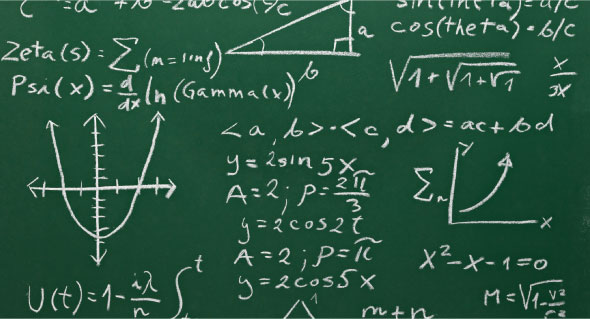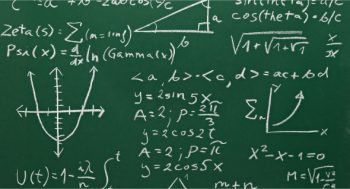# Lists: The Building Blocks of Maxima

0
4722This 20th article in our series on Mathematics in Open Source showcases the list manipulations in Maxima, the programming language with an ALGOL-like syntax but Lisp-like semantics.

Lists are the basic building blocks of Maxima. The fundamental reason is that Maxima is implemented in Lisp, the building blocks of which are also lists.
To begin with, let us walk through the ways of creating a list. The simplest method to get a list in Maxima is to just define it, using []. So, [x, 5, 3, 2*y] is a list consisting of four members. However, Maxima provides two powerful functions for automatically generating lists: makelist() and create_list().
makelist() can take two forms. makelist (e, x, x0, xn) creates and returns a list using the expression e, evaluated for x using the values ranging from x0 to xn. makelist(e, x, L) creates and returns a list using the expression e, evaluated for x using the members of the list L. Check out the example below for better clarity:

```\$ maxima -q
(%i1) makelist(2 * i, i, 1, 5);
(%o1) [2, 4, 6, 8, 10]
(%i2) makelist(concat(x, 2 * i - 1), i, 1, 5);
(%o2) [x1, x3, x5, x7, x9]
(%i3) makelist(concat(x, 2), x, [a, b, c, d]);
(%o3) [a2, b2, c2, d2]
(%i4) quit();```

Note the interesting usage of concat() to just concatenate its arguments. Note that makelist() is limited by the variation it can have, which to be specific, is just one  i in the first two examples and x in the last one. If we want more, the create_list() function comes into play.
create_list(f, x1, L1, …, xn, Ln) creates and returns a list with members of the form f, evaluated for the variables x1, …, xn using the values from the corresponding lists L1, …, Ln. Here is just a glimpse of its power:

```\$ maxima -q
(%i1) create_list(concat(x, y), x, [p, q], y, [1, 2]);
(%o1) [p1, p2, q1, q2]
(%i2) create_list(concat(x, y, z), x, [p, q], y, [1, 2], z, [a, b]);
(%o2) [p1a, p1b, p2a, p2b, q1a, q1b, q2a, q2b]
(%i3) create_list(concat(x, y, z), x, [p, q], y, [1, 2, 3], z, [a, b]);
(%o3) [p1a, p1b, p2a, p2b, p3a, p3b, q1a, q1b, q2a, q2b, q3a, q3b]
(%i4) quit();```

Note that all possible combinations are created using the values for the variables x, y and z.
Once we have created the lists, Maxima provides a host of functions to play around with them. Lets take a look at these.
Testing the lists
The following set of functions demonstrates the various checks on lists:

• atom(v) – returns true if v is an atomic element; false otherwise
• listp(L) – returns true if L is a list; false otherwise
• member(v, L) – returns true if v is a member of list L; false otherwise
• some(p, L) – returns true if predicate p is true for at least one member of list L; false otherwise
• every(p, L) – returns true if predicate p is true for all members of list L; false otherwise
```\$ maxima -q
(%i1) atom(5);
(%o1) true
(%i2) atom();
(%o2) false
(%i3) listp(x);
(%o3) false
(%i4) listp([x]);
(%o4) true
(%i5) listp([x, 5]);
(%o5) true
(%i6) member(x, [a, b, c]);
(%o6) false
(%i7) member(x, [a, x, c]);
(%o7) true
(%i8) some(primep, [1, 4, 9]);
(%o8) false
(%i9) some(primep, [1, 2, 4, 9]);
(%o9) true
(%i10) every(integerp, [1, 2, 4, 9]);
(%o10) true
(%i11) every(integerp, [1, 2, 4, x]);
(%o11) false
(%i12) quit();```

List recreations
Next is a set of functions operating on list(s) to create and return new lists:

• cons(v, L) – returns a list with v, followed by members of L
• endcons(v, L) – returns a list with members of L followed by v
• rest(L, n) – returns a list with members of L, except the first n members (if n is non-negative), otherwise except the last -n members. n is optional, in which case, it is taken as 1
• join(L1, L2) – returns a list with members of L1 and L2 interspersed
• delete(v, L, n) – returns a list like L but with the first n occurrences of v deleted from it. n is optional, in which case all occurrences of v are deleted
• append(L1, …, Ln) – returns a list with members of L1, …, Ln, one after the other
• unique(L) – returns a list obtained by removing the duplicate members in the list L
• reverse(L) – returns a list with members of the list L in reverse order
```\$ maxima -q
(%i1) L: makelist(i, i, 1, 10);
(%o1) [1, 2, 3, 4, 5, 6, 7, 8, 9, 10]
(%i2) cons(0, L);
(%o2) [0, 1, 2, 3, 4, 5, 6, 7, 8, 9, 10]
(%i3) endcons(11, L);
(%o3) [1, 2, 3, 4, 5, 6, 7, 8, 9, 10, 11]
(%i4) rest(L);
(%o4) [2, 3, 4, 5, 6, 7, 8, 9, 10]
(%i5) rest(L, 3);
(%o5) [4, 5, 6, 7, 8, 9, 10]
(%i6) rest(L, -3);
(%o6) [1, 2, 3, 4, 5, 6, 7]
(%i7) join(L, [a, b, c, d]);
(%o7) [1, a, 2, b, 3, c, 4, d]
(%i8) delete(6, L);
(%o8) [1, 2, 3, 4, 5, 7, 8, 9, 10]
(%i9) delete(4, delete(6, L));
(%o9) [1, 2, 3, 5, 7, 8, 9, 10]
(%i10) delete(4, delete(6, join(L, L)));
(%o10) [1, 1, 2, 2, 3, 3, 5, 5, 7, 7, 8, 8, 9, 9, 10, 10]
(%i11) L1: rest(L, 7);
(%o11) [8, 9, 10]
(%i12) L2: rest(rest(L, -3), 3);
(%o12) [4, 5, 6, 7]
(%i13) L3: rest(L, -7);
(%o13) [1, 2, 3]
(%i14) append(L1, L2, L3);
(%o14) [8, 9, 10, 4, 5, 6, 7, 1, 2, 3]
(%i15) reverse(L);
(%o15) [10, 9, 8, 7, 6, 5, 4, 3, 2, 1]
(%i16) join(reverse(L), L);
(%o16) [10, 1, 9, 2, 8, 3, 7, 4, 6, 5, 5, 6, 4, 7, 3, 8, 2, 9, 1, 10]
(%i17) unique(join(reverse(L), L));
(%o17) [1, 2, 3, 4, 5, 6, 7, 8, 9, 10]
(%i18) L;
(%o18) [1, 2, 3, 4, 5, 6, 7, 8, 9, 10]
(%i19) quit();```

Note that the list L is still not modified. For that matter, , even L1, L2, L3 are not modified. In fact, that is what is meant when we state that all these functions recreate new modified lists, rather than modify the existing ones.

List extractions
Here is a set of functions extracting the various members of a list. first(L), second(L), third(L), fourth(L), fifth(L), sixth(L), seventh(L), eight(L), ninth(L), and tenth(L), respectively return the first, second, … member of the list L. last(L) returns the last member of the list L.

```\$ maxima -q
(%i1) L: create_list(i * x, x, [a, b, c], i, [1, 2, 3, 4]);
(%o1) [a, 2 a, 3 a, 4 a, b, 2 b, 3 b, 4 b, c, 2 c, 3 c, 4 c]
(%i2) first(L);
(%o2) a
(%i3) seventh(L);
(%o3) 3 b
(%i4) last(L);
(%o4) 4 c
(%i5) third(L); last(L);
(%o5) 3 a
(%o6) 4 c
(%i7) L;
(%o7) [a, 2 a, 3 a, 4 a, b, 2 b, 3 b, 4 b, c, 2 c, 3 c, 4 c]
(%i8) quit();```

Again, note that the list L is still not modified. However, we may need to modify the existing lists, and none of the above functions will do that. It could be achieved by assigning the return values of the various list recreation functions back to the original list. However, there are a few functions, which do modify the list right away.
List manipulations
The following are the two list manipulating functions provided by Maxima:

• push(v, L) – inserts v at the beginning of the list L
• pop(L) – removes and returns the first element from list L

L must be a symbol bound to a list, not the list itself, in both the above functions, for them to modify it. Also, these functionalities are not available by default, so we need to load the basic Maxima file. Check out the demonstration below.
We may display L after doing these operations, or even check the length of L to verify the actual modification of L. In case we need to preserve a copy of the list, the function copylist() can be used.

```\$ maxima -q
(%i1) L: makelist(2 * x, x, 1, 10);
(%o1) [2, 4, 6, 8, 10, 12, 14, 16, 18, 20]
(%i2) push(0, L); /* This doesnt work */
(%o2) push(0, [2, 4, 6, 8, 10, 12, 14, 16, 18, 20])
(%i3) pop(L); /* Nor does this work */
(%o3) pop([2, 4, 6, 8, 10, 12, 14, 16, 18, 20])
(%o4) /usr/share/maxima/5.24.0/share/macro/basic.mac
(%i5) push(0, L); /* Now, this works */
(%o5) [0, 2, 4, 6, 8, 10, 12, 14, 16, 18, 20]
(%i6) L;
(%o6) [0, 2, 4, 6, 8, 10, 12, 14, 16, 18, 20]
(%i7) pop(L); /* Even this works */
(%o7) 0
(%i8) L;
(%o8) [2, 4, 6, 8, 10, 12, 14, 16, 18, 20]
(%i9) K: copylist(L);
(%o9) [2, 4, 6, 8, 10, 12, 14, 16, 18, 20]
(%i10) length(L);
(%o10) 10
(%i11) pop(L);
(%o11) 2
(%i12) length(L);
(%o12) 9
(%i13) K;
(%o13) [2, 4, 6, 8, 10, 12, 14, 16, 18, 20]
(%i14) L;
(%o14) [4, 6, 8, 10, 12, 14, 16, 18, 20]
(%i15) pop([1, 2, 3]); /* Actual list is not allowed */
arg must be a symbol [1, 2, 3]
#0: symbolcheck(x=[1,2,3])(basic.mac line 22)
#1: pop(l=[1,2,3])(basic.mac line 26)
-- an error. To debug this try: debugmode(true);
(%i16) quit();```

And finally, here is a bonus of two sophisticated list operations:

• sublist_indices(L, p) – returns the list indices for the members of the list L, for which predicate p is true.
• assoc(k, L, d) – L must have all its members in the form of x op y, where op is some binary operator. Then, assoc() searches for k in the left operand of the members of L. If found, it returns the corresponding right operand, otherwise it returnsd; or it returns false, if d is missing.

Check out the demonstration below for both the above operations

```\$ maxima -q
(%i1) sublist_indices([12, 23, 57, 37, 64, 67], primep);
(%o1) [2, 4, 6]
(%i2) sublist_indices([12, 23, 57, 37, 64, 67], evenp);
(%o2) [1, 5]
(%i3) sublist_indices([12, 23, 57, 37, 64, 67], oddp);
(%o3) [2, 3, 4, 6]
(%i4) sublist_indices([2 > 0, -2 > 0, 1 = 1, x = y], identity);
(%o4) [1, 3]
(%i5) assoc(2, [2^r, x+y, 2=4, 5/6]);
(%o5) r
(%i6) assoc(6, [2^r, x+y, 2=4, 5/6]);
(%o6) false
(%i7) assoc(6, [2^r, x+y, 2=4, 5/6], na);
(%o7) na
(%i8) quit();```